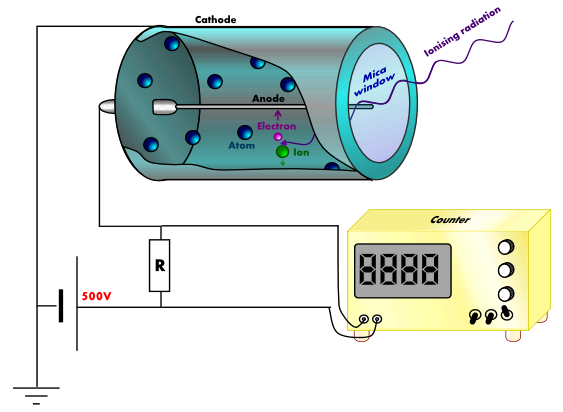# 19.03: Instruments for Radiation Detection

Because radiation is harmful to humans and other organisms, it is very important that we be able to detect it and measure how much is present. Such measurements are complicated by two factors. First, we cannot see, hear, smell, taste, or touch radiation, and so special instruments are required to measure it. Second, different types of radiation are more dangerous than others, and corrections must be made for the relative harm done by α particles as opposed to, say, γ rays.Figure $$\PageIndex{1}$$: A Geiger-Müller tube.

Perhaps the most common instrument for measuring radiation levels is the Geiger-Müller counter (the same Geiger who worked with Rutherford to discover the atomic nucleus). A schematic diagram of a Geiger-Müller counter is shown in Fig. $$\PageIndex{1}$$ . A metal tube containing Ar gas is sealed at one end with a thin glass or plastic window and contains a central wire well insulated from it. A potential difference of about 1000 V is applied between the central wire and the tube. Any incoming α, β or γ ray will ionize some of the Ar atoms. These Ar+ ions are quickly accelerated to a high velocity by the large potential difference, high enough for them in turn to start ionizing further Ar atoms. Thus, for every ray that enters the tube, a large number of ions is formed and a pulse of electrical current is produced. This pulse is amplified and allowed to drive a digital electronic counter which operates on a principle similar to that of a digital watch. The number of particles passing through the tube in a given time can thus be found. Alternatively, the tube can be made to operate a meter indicating the rate at which radiation is passing into the Geiger-Müller tube.

Another type of detector, much used for γ rays, is the scintillation counter. When a γ ray penetrates a special crystal or solution, it produces a momentary flash of light (called a scintillation) which is detected by a photoelectric cell. Again the output can be amplified and fed into a counter or a meter. A third kind of detector is used to monitor how much exposure laboratory workers have been subjected to in the course of their work. This is simply a strip of photographic film. The degree to which this film is darkened is a measure of the total quantity of radiation to which the worker has been subjected.

A variety of units are used to measure various aspects of radiation (Table $$\PageIndex{1}$$). The SI unit for rate of radioactive decay is the becquerel (Bq), with 1 Bq = 1 disintegration per second. The curie (Ci) and millicurie (mCi) are much larger units and are frequently used in medicine (1 curie = 1 Ci = $$3.7 \times 10^{10}$$ disintegrations per second). The SI unit for measuring radiation dose is the gray (Gy), with 1 Gy = 1 J of energy absorbed per kilogram of tissue. In medical applications, the radiation absorbed dose (rad) is more often used (1 rad = 0.01 Gy; 1 rad results in the absorption of 0.01 J/kg of tissue). The SI unit measuring tissue damage caused by radiation is the sievert (Sv). This takes into account both the energy and the biological effects of the type of radiation involved in the radiation dose.

Table $$\PageIndex{1}$$: Units Used for Measuring Radiation
Measurement Purpose Unit Quantity Measured Description
activity of source becquerel (Bq) radioactive decays or emissions amount of sample that undergoes 1 decay/second
curie (Ci) amount of sample that undergoes $$\mathrm{3.7 \times 10^{10}\; decays/second}$$
absorbed dose gray (Gy) energy absorbed per kg of tissue 1 Gy = 1 J/kg tissue
biologically effective dose sievert (Sv) tissue damage Sv = RBE × Gy
roentgen equivalent for man (rem) Rem = RBE × rad

The roentgen equivalent for man (rem) is the unit for radiation damage that is used most frequently in medicine (1 rem = 1 Sv). Note that the tissue damage units (rem or Sv) includes the energy of the radiation dose (rad or Gy) along with a biological factor referred to as the RBE (for relative biological effectiveness) that is an approximate measure of the relative damage done by the radiation. These are related by:

$\text{number of rems}=\text{RBE} \times \text{number of rads} \label{Eq2}$

with RBE approximately 10 for α radiation, 2(+) for protons and neutrons, and 1 for β and γ radiation.Figure $$\PageIndex{7}$$: Different units are used to measure the rate of emission from a radioactive source, the energy that is absorbed from the source, and the amount of damage the absorbed radiation does.